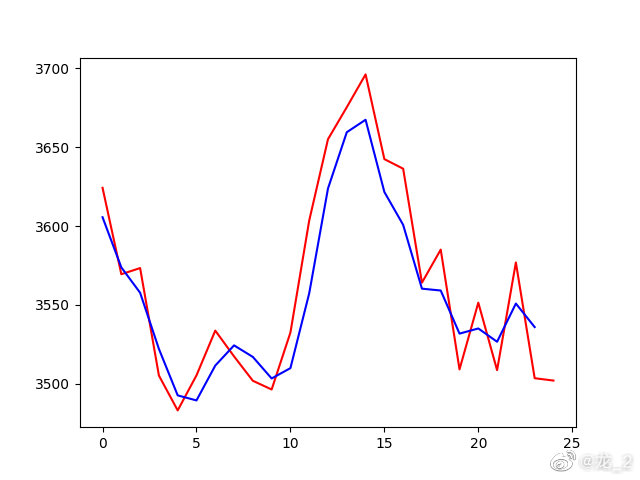使用循环神经网络预测股价

1. 获得训练数据

pip install pandas
pip install tensorflow==2.3.0

Tushare的使用非常简单，直接调用获取数据的方法即可返回数据集，需要传入的参数包括股票的代码，上证综合指数为sh000001，以及获取数据的日期区间。同时将数据按照时间索引进行排序，获取数据的代码如下

data = tushare.get_hist_data('sh000001', '2011-01-01', '2020-12-31');
data.index = data.index.astype('datetime64[ns]');
data = data.sort_index()['close'].values.reshape(-1, 1);

2. 生成训练数据集

#归一化处理
scl = MinMaxScaler();
data = scl.fit_transform(data);
#预测使用的数据点个数及预测的数据点个数
look_back = 30;
forward_days = 1;
#生成训练数据集
x_train, y_train = [], [];
for i in range(0, len(data) - look_back - forward_days + 1):
x_train.append(data[i : (i + look_back)]);
y_train.append(data[(i + look_back) : (i + look_back + forward_days)]);
x_train = numpy.array(x_train);
y_train = numpy.array(y_train);

3. 构造循环神经网络模型

#初始化循环神经网络模型
model = Sequential();
#第一层长短期记忆层
#防止过拟合增加Dropout层
#第二层长短期记忆层
#最后的全连接层
#编译模型
#进行数据拟合
model.fit(x_train, y_train, epochs=100, batch_size=16);
#保存训练得到的模型
model.save(model_file);

import os;
os.environ['CUDA_VISIBLE_DEVICES'] = '-1';

4. 进行预测

#获取预测需要的数据，并对数据进行处理
data = tushare.get_hist_data(no, '2021-01-01');
data.index = data.index.astype('datetime64[ns]');
data = data.sort_index()['close'].values.reshape(-1, 1);
data = scl.fit_transform(data);
#构造预测的输入数据集
x_test = [];
for i in range(0, len(data) - look_back - forward_days + 1):
x_test.append(data[i : (i + look_back)]);
x_test = numpy.array(x_test);
#使用模型对结果进行预测
y_test = model.predict(x_test);

import matplotlib.pyplot as plt;
plt.plot(scl.inverse_transform(y_test.reshape(-1, 1))[0:-1]);
plt.plot(scl.inverse_transform(data[look_back + 1:]));
plt.show();

5.结果